# secml.ml.classifiers.loss¶

## CLoss¶

class secml.ml.classifiers.loss.c_loss.CLoss[source]

Interface for loss functions.

Attributes
class_type

Defines class type.

logger

Logger for current object.

suitable_for

Defines which problem the loss is suitable for.

verbose

Verbosity level of logger output.

Methods

 copy(self) Returns a shallow copy of current class. create([class_item]) This method creates an instance of a class with given type. deepcopy(self) Returns a deep copy of current class. dloss(self, y_true, score) Computes the derivative of the loss function with respect to score. get_class_from_type(class_type) Return the class associated with input type. get_params(self) Returns the dictionary of class parameters. get_state(self) Returns the object state dictionary. get_subclasses() Get all the subclasses of the calling class. list_class_types() This method lists all types of available subclasses of calling one. load(path) Loads object from file. load_state(self, path) Sets the object state from file. loss(self, y_true, score) Computes the value of the loss function. save(self, path) Save class object to file. save_state(self, path) Store the object state to file. set(self, param_name, param_value[, copy]) Set a parameter of the class. set_params(self, params_dict[, copy]) Set all parameters passed as a dictionary {key: value}. set_state(self, state_dict[, copy]) Sets the object state using input dictionary. timed([msg]) Timer decorator.
dloss(self, y_true, score)[source]

Computes the derivative of the loss function with respect to score.

Parameters
y_trueCArray

Ground truth (correct), targets. Vector-like array.

scoreCArray

Outputs (predicted), targets.

Returns
CArray

Derivative of the loss function. Vector-like array.

abstract loss(self, y_true, score)[source]

Computes the value of the loss function.

Parameters
y_trueCArray

Ground truth (correct), targets. Vector-like array.

scoreCArray

Outputs (predicted), targets.

Returns
CArray

Loss function. Vector-like array.

abstract property suitable_for

Defines which problem the loss is suitable for.

Accepted values: - classification - regression

class secml.ml.classifiers.loss.c_loss.CLossClassification[source]

Interface for loss functions suitable for classification problems.

Attributes
class_type

Defines class type.

logger

Logger for current object.

verbose

Verbosity level of logger output.

Methods

 copy(self) Returns a shallow copy of current class. create([class_item]) This method creates an instance of a class with given type. deepcopy(self) Returns a deep copy of current class. dloss(self, y_true, score[, pos_label]) Computes the derivative of the loss function with respect to score. get_class_from_type(class_type) Return the class associated with input type. get_params(self) Returns the dictionary of class parameters. get_state(self) Returns the object state dictionary. get_subclasses() Get all the subclasses of the calling class. list_class_types() This method lists all types of available subclasses of calling one. load(path) Loads object from file. load_state(self, path) Sets the object state from file. loss(self, y_true, score[, pos_label]) Computes the value of the loss function. save(self, path) Save class object to file. save_state(self, path) Store the object state to file. set(self, param_name, param_value[, copy]) Set a parameter of the class. set_params(self, params_dict[, copy]) Set all parameters passed as a dictionary {key: value}. set_state(self, state_dict[, copy]) Sets the object state using input dictionary. timed([msg]) Timer decorator.
dloss(self, y_true, score, pos_label=None)[source]

Computes the derivative of the loss function with respect to score.

Parameters
y_trueCArray

Ground truth (correct), targets. Vector-like array.

scoreCArray

Outputs (predicted), targets. 2-D array of shape (n_samples, n_classes) or 1-D flat array of shape (n_samples,).

pos_labelint or None, optional

Default None, meaning that the function derivative is computed for each sample wrt the corresponding true label. Otherwise, this is the class wrt compute the derivative. If score is a 1-D flat array, this parameter is ignored.

Returns
CArray

Derivative of the loss function. Vector-like array.

abstract loss(self, y_true, score, pos_label=None)[source]

Computes the value of the loss function.

Parameters
y_trueCArray

Ground truth (correct), targets. Vector-like array.

scoreCArray

Outputs (predicted), targets. 2-D array of shape (n_samples, n_classes) or 1-D flat array of shape (n_samples,).

pos_labelint or None, optional

Default None, meaning that the function is computed for each sample wrt the corresponding true label. Otherwise, this is the class wrt compute the loss function. If score is a 1-D flat array, this parameter is ignored.

Returns
CArray

Loss function. Vector-like array.

suitable_for = 'classification'
class secml.ml.classifiers.loss.c_loss.CLossRegression[source]

Interface for loss functions suitable for regression problems.

Attributes
class_type

Defines class type.

logger

Logger for current object.

verbose

Verbosity level of logger output.

Methods

 copy(self) Returns a shallow copy of current class. create([class_item]) This method creates an instance of a class with given type. deepcopy(self) Returns a deep copy of current class. dloss(self, y_true, score) Computes the derivative of the loss function with respect to score. get_class_from_type(class_type) Return the class associated with input type. get_params(self) Returns the dictionary of class parameters. get_state(self) Returns the object state dictionary. get_subclasses() Get all the subclasses of the calling class. list_class_types() This method lists all types of available subclasses of calling one. load(path) Loads object from file. load_state(self, path) Sets the object state from file. loss(self, y_true, score) Computes the value of the loss function. save(self, path) Save class object to file. save_state(self, path) Store the object state to file. set(self, param_name, param_value[, copy]) Set a parameter of the class. set_params(self, params_dict[, copy]) Set all parameters passed as a dictionary {key: value}. set_state(self, state_dict[, copy]) Sets the object state using input dictionary. timed([msg]) Timer decorator.
abstract dloss(self, y_true, score)[source]

Computes the derivative of the loss function with respect to score.

Parameters
y_trueCArray

Ground truth (correct), targets. Vector-like array.

scoreCArray

Outputs (predicted), targets. Vector-like array of shape (n_samples,).

Returns
CArray

Derivative of the loss function. Vector-like array.

abstract loss(self, y_true, score)[source]

Computes the value of the loss function.

Parameters
y_trueCArray

Ground truth (correct), targets. Vector-like array.

scoreCArray

Outputs (predicted), targets. Vector-like array of shape (n_samples,).

Returns
CArray

Loss function. Vector-like array.

suitable_for = 'regression'

## CLossCrossEntropy¶

class secml.ml.classifiers.loss.c_loss_cross_entropy.CLossCrossEntropy[source]

Cross Entropy Loss Function (Log Loss).

Cross entropy indicates the distance between what the model believes the output distribution should be, and what the original distribution really is.

The cross entropy loss is defined as (for sample i):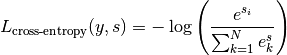Attributes
class_type‘cross-entropy’

Defines class type.

suitable_for‘classification’

Methods

 copy(self) Returns a shallow copy of current class. create([class_item]) This method creates an instance of a class with given type. deepcopy(self) Returns a deep copy of current class. dloss(self, y_true, score[, pos_label]) Computes gradient of the Cross Entropy loss w.r.t.the classifier get_class_from_type(class_type) Return the class associated with input type. get_params(self) Returns the dictionary of class parameters. get_state(self) Returns the object state dictionary. get_subclasses() Get all the subclasses of the calling class. list_class_types() This method lists all types of available subclasses of calling one. load(path) Loads object from file. load_state(self, path) Sets the object state from file. loss(self, y_true, score[, pos_label]) Computes the value of the Cross Entropy loss function. save(self, path) Save class object to file. save_state(self, path) Store the object state to file. set(self, param_name, param_value[, copy]) Set a parameter of the class. set_params(self, params_dict[, copy]) Set all parameters passed as a dictionary {key: value}. set_state(self, state_dict[, copy]) Sets the object state using input dictionary. timed([msg]) Timer decorator.
dloss(self, y_true, score, pos_label=None)[source]
Computes gradient of the Cross Entropy loss w.r.t.the classifier

decision function corresponding to class label pos_label.

Assuming pos_label to be i, the derivative is:

p_i - t_i, t_i = 1 if i is equal to y_true_i, 0 otherwise

Then, the elements corresponding to y_true (if pos_label is None) or pos_label will be returned.

Parameters
y_trueCArray

Ground truth (correct), targets. Vector-like array.

scoreCArray

Outputs (predicted), targets. 2-D array of shape (n_samples, n_classes).

pos_labelint or None, optional

The class wrt compute the loss function. Default None, meaning that the function is computed for each sample wrt the corresponding true label.

Returns
CArray

Loss function. Vector-like array.

loss(self, y_true, score, pos_label=<no value>)[source]

Computes the value of the Cross Entropy loss function.

Parameters
y_trueCArray

Ground truth (correct), targets. Vector-like array.

scoreCArray

Outputs (predicted), targets. 2-D array of shape (n_samples, n_classes).

Returns
CArray

Loss function. Vector-like array.

Notes

Differently from other loss functions, CrossEntropyLoss requires the full array (n_samples, n_classes) of predicted outputs.

## CLossEpsilonInsensitive¶

class secml.ml.classifiers.loss.c_loss_epsilon_insensitive.CLossEpsilonInsensitive(epsilon=0.1)[source]

Epsilon-Insensitive Loss Function.

Any difference between the current prediction and the ground truth is ignored if is less than the epsilon threshold.

Epsilon-Insensitive loss is used by support vector regression.

The Epsilon-Insensitive loss is defined as: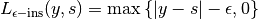Attributes
class_type‘e-insensitive’

Defines class type.

suitable_for‘regression’

Methods

 copy(self) Returns a shallow copy of current class. create([class_item]) This method creates an instance of a class with given type. deepcopy(self) Returns a deep copy of current class. dloss(self, y_true, score) Computes the derivative of the epsilon-insensitive loss function get_class_from_type(class_type) Return the class associated with input type. get_params(self) Returns the dictionary of class parameters. get_state(self) Returns the object state dictionary. get_subclasses() Get all the subclasses of the calling class. list_class_types() This method lists all types of available subclasses of calling one. load(path) Loads object from file. load_state(self, path) Sets the object state from file. loss(self, y_true, score) Computes the value of the epsilon-insensitive loss function. save(self, path) Save class object to file. save_state(self, path) Store the object state to file. set(self, param_name, param_value[, copy]) Set a parameter of the class. set_params(self, params_dict[, copy]) Set all parameters passed as a dictionary {key: value}. set_state(self, state_dict[, copy]) Sets the object state using input dictionary. timed([msg]) Timer decorator.
dloss(self, y_true, score)[source]
Computes the derivative of the epsilon-insensitive loss function

with respect to score.

Parameters
y_trueCArray

Ground truth (correct), targets. Vector-like array.

scoreCArray

Outputs (predicted), targets. Vector-like array of shape (n_samples,).

Returns
CArray

Derivative of the loss function. Vector-like array.

property epsilon

Threshold parameter epsilon.

loss(self, y_true, score)[source]

Computes the value of the epsilon-insensitive loss function.

Parameters
y_trueCArray

Ground truth (correct), targets. Vector-like array.

scoreCArray

Outputs (predicted), targets. Vector-like array of shape (n_samples,).

Returns
CArray

Loss function. Vector-like array.

class secml.ml.classifiers.loss.c_loss_epsilon_insensitive.CLossEpsilonInsensitiveSquared(epsilon=0.1)[source]

Squared Epsilon-Insensitive Loss Function.

Any difference between the current prediction and the ground truth is ignored if is less than the epsilon threshold.

The Squared Epsilon-Insensitive loss is defined as: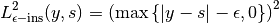Attributes
class_type‘e-insensitive-squared’

Defines class type.

suitable_for‘regression’

Methods

 copy(self) Returns a shallow copy of current class. create([class_item]) This method creates an instance of a class with given type. deepcopy(self) Returns a deep copy of current class. dloss(self, y_true, score) Computes the derivative of the squared epsilon-insensitive get_class_from_type(class_type) Return the class associated with input type. get_params(self) Returns the dictionary of class parameters. get_state(self) Returns the object state dictionary. get_subclasses() Get all the subclasses of the calling class. list_class_types() This method lists all types of available subclasses of calling one. load(path) Loads object from file. load_state(self, path) Sets the object state from file. loss(self, y_true, score) Computes the value of the squared epsilon-insensitive loss function. save(self, path) Save class object to file. save_state(self, path) Store the object state to file. set(self, param_name, param_value[, copy]) Set a parameter of the class. set_params(self, params_dict[, copy]) Set all parameters passed as a dictionary {key: value}. set_state(self, state_dict[, copy]) Sets the object state using input dictionary. timed([msg]) Timer decorator.
dloss(self, y_true, score)[source]
Computes the derivative of the squared epsilon-insensitive

loss function with respect to score.

Parameters
y_trueCArray

Ground truth (correct), targets. Vector-like array.

scoreCArray

Outputs (predicted), targets. Vector-like array of shape (n_samples,).

Returns
CArray

Derivative of the loss function. Vector-like array.

loss(self, y_true, score)[source]

Computes the value of the squared epsilon-insensitive loss function.

Parameters
y_trueCArray

Ground truth (correct), targets. Vector-like array.

scoreCArray

Outputs (predicted), targets. Vector-like array of shape (n_samples,).

Returns
CArray

Loss function. Vector-like array.

## CLossHinge¶

class secml.ml.classifiers.loss.c_loss_hinge.CLossHinge[source]

Hinge Loss Function.

The function computes the average distance between the model and

the data using hinge loss, a one-sided metric that considers only prediction errors.

Hinge loss is used in maximal margin classifiers such as

support vector machines.

After converting the labels to {-1, +1},

then the hinge loss is defined as: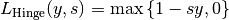Attributes
class_type‘hinge’

Defines class type.

suitable_for‘classification’

Methods

 copy(self) Returns a shallow copy of current class. create([class_item]) This method creates an instance of a class with given type. deepcopy(self) Returns a deep copy of current class. dloss(self, y_true, score[, pos_label]) Computes the derivative of the hinge loss function with respect to score. get_class_from_type(class_type) Return the class associated with input type. get_params(self) Returns the dictionary of class parameters. get_state(self) Returns the object state dictionary. get_subclasses() Get all the subclasses of the calling class. list_class_types() This method lists all types of available subclasses of calling one. load(path) Loads object from file. load_state(self, path) Sets the object state from file. loss(self, y_true, score[, pos_label]) Computes the value of the hinge loss function. save(self, path) Save class object to file. save_state(self, path) Store the object state to file. set(self, param_name, param_value[, copy]) Set a parameter of the class. set_params(self, params_dict[, copy]) Set all parameters passed as a dictionary {key: value}. set_state(self, state_dict[, copy]) Sets the object state using input dictionary. timed([msg]) Timer decorator.
dloss(self, y_true, score, pos_label=1)[source]

Computes the derivative of the hinge loss function with respect to score.

Parameters
y_trueCArray

Ground truth (correct), targets. Vector-like array.

scoreCArray

Outputs (predicted), targets. 2-D array of shape (n_samples, n_classes) or 1-D flat array of shape (n_samples,). If 1-D array, the probabilities provided are assumed to be that of the positive class.

pos_label{0, 1}, optional

The class wrt compute the loss function derivative. Default 1. If score is a 1-D flat array, this parameter is ignored.

Returns
CArray

Derivative of the loss function. Vector-like array.

loss(self, y_true, score, pos_label=1)[source]

Computes the value of the hinge loss function.

Parameters
y_trueCArray

Ground truth (correct), targets. Vector-like array.

scoreCArray

Outputs (predicted), targets. 2-D array of shape (n_samples, n_classes) or 1-D flat array of shape (n_samples,). If 1-D array, the probabilities provided are assumed to be that of the positive class.

pos_label{0, 1}, optional

The class wrt compute the loss function. Default 1. If score is a 1-D flat array, this parameter is ignored.

Returns
CArray

Loss function. Vector-like array.

class secml.ml.classifiers.loss.c_loss_hinge.CLossHingeSquared[source]

Squared Hinge Loss Function.

The function computes the average distance between the model and the data using hinge loss, a one-sided metric that considers only prediction errors.

After converting the labels to {-1, +1}, then the hinge loss is defined as:Attributes
class_type‘hinge-squared’

Defines class type.

suitable_for‘classification’

Methods

 copy(self) Returns a shallow copy of current class. create([class_item]) This method creates an instance of a class with given type. deepcopy(self) Returns a deep copy of current class. dloss(self, y_true, score[, pos_label]) Computes the derivative of the squared hinge loss function with respect to score. get_class_from_type(class_type) Return the class associated with input type. get_params(self) Returns the dictionary of class parameters. get_state(self) Returns the object state dictionary. get_subclasses() Get all the subclasses of the calling class. list_class_types() This method lists all types of available subclasses of calling one. load(path) Loads object from file. load_state(self, path) Sets the object state from file. loss(self, y_true, score[, pos_label]) Computes the value of the squared hinge loss function. save(self, path) Save class object to file. save_state(self, path) Store the object state to file. set(self, param_name, param_value[, copy]) Set a parameter of the class. set_params(self, params_dict[, copy]) Set all parameters passed as a dictionary {key: value}. set_state(self, state_dict[, copy]) Sets the object state using input dictionary. timed([msg]) Timer decorator.
dloss(self, y_true, score, pos_label=1)[source]

Computes the derivative of the squared hinge loss function with respect to score.

Parameters
y_trueCArray

Ground truth (correct), targets. Vector-like array.

scoreCArray

Outputs (predicted), targets. 2-D array of shape (n_samples, n_classes) or 1-D flat array of shape (n_samples,). If 1-D array, the probabilities provided are assumed to be that of the positive class.

pos_label{0, 1}, optional

The class wrt compute the loss function derivative. Default 1. If score is a 1-D flat array, this parameter is ignored.

Returns
CArray

Derivative of the loss function. Vector-like array.

loss(self, y_true, score, pos_label=1)[source]

Computes the value of the squared hinge loss function.

Parameters
y_trueCArray

Ground truth (correct), targets. Vector-like array.

scoreCArray

Outputs (predicted), targets. 2-D array of shape (n_samples, n_classes) or 1-D flat array of shape (n_samples,). If 1-D array, the probabilities provided are assumed to be that of the positive class.

pos_label{0, 1}, optional

The class wrt compute the loss function. Default 1. If score is a 1-D flat array, this parameter is ignored.

Returns
CArray

Loss function. Vector-like array.

## CLossLogistic¶

class secml.ml.classifiers.loss.c_loss_logistic.CLossLogistic[source]

Logistic loss function.

Attributes
class_type‘log’

Defines class type.

suitable_for‘classification’

Methods

 copy(self) Returns a shallow copy of current class. create([class_item]) This method creates an instance of a class with given type. deepcopy(self) Returns a deep copy of current class. dloss(self, y_true, score[, pos_label, bound]) Computes the derivative of the hinge loss function with respect to score. get_class_from_type(class_type) Return the class associated with input type. get_params(self) Returns the dictionary of class parameters. get_state(self) Returns the object state dictionary. get_subclasses() Get all the subclasses of the calling class. list_class_types() This method lists all types of available subclasses of calling one. load(path) Loads object from file. load_state(self, path) Sets the object state from file. loss(self, y_true, score[, pos_label, bound]) Computes the value of the logistic loss function. save(self, path) Save class object to file. save_state(self, path) Store the object state to file. set(self, param_name, param_value[, copy]) Set a parameter of the class. set_params(self, params_dict[, copy]) Set all parameters passed as a dictionary {key: value}. set_state(self, state_dict[, copy]) Sets the object state using input dictionary. timed([msg]) Timer decorator.
dloss(self, y_true, score, pos_label=1, bound=10)[source]

Computes the derivative of the hinge loss function with respect to score.

Parameters
y_trueCArray

Ground truth (correct), targets. Vector-like array.

scoreCArray

Outputs (predicted), targets. 2-D array of shape (n_samples, n_classes) or 1-D flat array of shape (n_samples,). If 1-D array, the probabilities provided are assumed to be that of the positive class.

pos_label{0, 1}, optional

The class wrt compute the loss function derivative. Default 1. If score is a 1-D flat array, this parameter is ignored.

boundscalar or None, optional

Set an upper bound for a linear approximation when -y*s is large to avoid numerical overflows. 10 is a generally acceptable -> log(1+exp(10)) = 10.000045

Returns
CArray

Derivative of the loss function. Vector-like array.

loss(self, y_true, score, pos_label=1, bound=10)[source]

Computes the value of the logistic loss function.

Parameters
y_trueCArray

Ground truth (correct), targets. Vector-like array.

scoreCArray

Outputs (predicted), targets. 2-D array of shape (n_samples, n_classes) or 1-D flat array of shape (n_samples,). If 1-D array, the probabilities provided are assumed to be that of the positive class.

pos_label{0, 1}, optional

The class wrt compute the loss function. Default 1. If score is a 1-D flat array, this parameter is ignored.

boundscalar or None, optional

Set an upper bound for a linear approximation when -y*s is large to avoid numerical overflows. 10 is a generally acceptable -> log(1+exp(10)) = 10.000045

Returns
CArray

Loss function. Vector-like array.

## CLossSquare¶

class secml.ml.classifiers.loss.c_loss_squared.CLossQuadratic[source]

Quadratic Loss Function (Ordinary Least Squares).

The quadratic loss is defined as: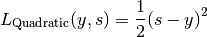Attributes
class_type‘quadratic’

Defines class type.

suitable_for‘regression’

Methods

 copy(self) Returns a shallow copy of current class. create([class_item]) This method creates an instance of a class with given type. deepcopy(self) Returns a deep copy of current class. dloss(self, y_true, score) Computes the derivative of the quadratic loss function with respect to score. get_class_from_type(class_type) Return the class associated with input type. get_params(self) Returns the dictionary of class parameters. get_state(self) Returns the object state dictionary. get_subclasses() Get all the subclasses of the calling class. list_class_types() This method lists all types of available subclasses of calling one. load(path) Loads object from file. load_state(self, path) Sets the object state from file. loss(self, y_true, score) Computes the value of the quadratic loss function. save(self, path) Save class object to file. save_state(self, path) Store the object state to file. set(self, param_name, param_value[, copy]) Set a parameter of the class. set_params(self, params_dict[, copy]) Set all parameters passed as a dictionary {key: value}. set_state(self, state_dict[, copy]) Sets the object state using input dictionary. timed([msg]) Timer decorator.
dloss(self, y_true, score)[source]

Computes the derivative of the quadratic loss function with respect to score.

Parameters
y_trueCArray

Ground truth (correct), targets. Vector-like array.

scoreCArray

Outputs (predicted), targets. Vector-like array of shape (n_samples,).

Returns
CArray

Derivative of the loss function. Vector-like array.

loss(self, y_true, score)[source]

Computes the value of the quadratic loss function.

Parameters
y_trueCArray

Ground truth (correct), targets. Vector-like array.

scoreCArray

Outputs (predicted), targets. Vector-like array of shape (n_samples,).

Returns
CArray

Loss function. Vector-like array.

class secml.ml.classifiers.loss.c_loss_squared.CLossSquare[source]

Square Loss Function.

The square loss is defined as: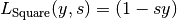Attributes
class_type‘square’

Defines class type.

suitable_for‘classification’

Methods

 copy(self) Returns a shallow copy of current class. create([class_item]) This method creates an instance of a class with given type. deepcopy(self) Returns a deep copy of current class. dloss(self, y_true, score[, pos_label]) Computes the derivative of the square loss function with respect to score. get_class_from_type(class_type) Return the class associated with input type. get_params(self) Returns the dictionary of class parameters. get_state(self) Returns the object state dictionary. get_subclasses() Get all the subclasses of the calling class. list_class_types() This method lists all types of available subclasses of calling one. load(path) Loads object from file. load_state(self, path) Sets the object state from file. loss(self, y_true, score[, pos_label]) Computes the value of the squared epsilon-insensitive loss function. save(self, path) Save class object to file. save_state(self, path) Store the object state to file. set(self, param_name, param_value[, copy]) Set a parameter of the class. set_params(self, params_dict[, copy]) Set all parameters passed as a dictionary {key: value}. set_state(self, state_dict[, copy]) Sets the object state using input dictionary. timed([msg]) Timer decorator.
dloss(self, y_true, score, pos_label=1)[source]

Computes the derivative of the square loss function with respect to score.

Parameters
y_trueCArray

Ground truth (correct), targets. Vector-like array.

scoreCArray

Outputs (predicted), targets. 2-D array of shape (n_samples, n_classes) or 1-D flat array of shape (n_samples,). If 1-D array, the probabilities provided are assumed to be that of the positive class.

pos_label{0, 1}, optional

The class wrt compute the loss function derivative. Default 1. If score is a 1-D flat array, this parameter is ignored.

Returns
CArray

Derivative of the loss function. Vector-like array.

loss(self, y_true, score, pos_label=1)[source]

Computes the value of the squared epsilon-insensitive loss function.

Parameters
y_trueCArray

Ground truth (correct), targets. Vector-like array.

scoreCArray

Outputs (predicted), targets. 2-D array of shape (n_samples, n_classes) or 1-D flat array of shape (n_samples,). If 1-D array, the probabilities provided are assumed to be that of the positive class.

pos_label{0, 1}, optional

The class wrt compute the loss function. Default 1. If score is a 1-D flat array, this parameter is ignored.

Returns
CArray

Loss function. Vector-like array.

## CSoftmax¶

class secml.ml.classifiers.loss.c_softmax.CSoftmax[source]

Softmax function.

Attributes
class_type

Defines class type.

logger

Logger for current object.

verbose

Verbosity level of logger output.

Methods

 copy(self) Returns a shallow copy of current class. create([class_item]) This method creates an instance of a class with given type. deepcopy(self) Returns a deep copy of current class. get_class_from_type(class_type) Return the class associated with input type. get_params(self) Returns the dictionary of class parameters. get_state(self) Returns the object state dictionary. get_subclasses() Get all the subclasses of the calling class. gradient(self, s, y) Gradient of the softmax function. list_class_types() This method lists all types of available subclasses of calling one. load(path) Loads object from file. load_state(self, path) Sets the object state from file. save(self, path) Save class object to file. save_state(self, path) Store the object state to file. set(self, param_name, param_value[, copy]) Set a parameter of the class. set_params(self, params_dict[, copy]) Set all parameters passed as a dictionary {key: value}. set_state(self, state_dict[, copy]) Sets the object state using input dictionary. softmax(self, s) Apply the softmax function to input. timed([msg]) Timer decorator.
gradient(self, s, y)[source]

The derivative of the y-th output of the softmax function w.r.t. all the inputs is given by: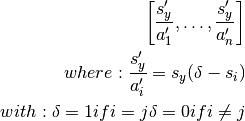Parameters
sCArray

2-D array of shape (1, n_classes) with input data.

yint

The class wrt compute the gradient.

Returns
CArray

softmax(self, s)[source]

Apply the softmax function to input.

The softmax function is defined for the vector s and for the i-th class as: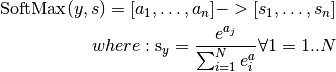Parameters
sCArray

2-D array of shape (n_samples, n_classes) with input data.

Returns
CArray

Softmax function. Same shape of input array.

Examples

>>> from secml.array import CArray
>>> from secml.ml.classifiers.loss import CSoftmax

>>> a = CArray([[1, 2, 3], [2, 4, 5]])
>>> print(CSoftmax().softmax(a))
CArray([[0.090031 0.244728 0.665241]
[0.035119 0.259496 0.705385]])